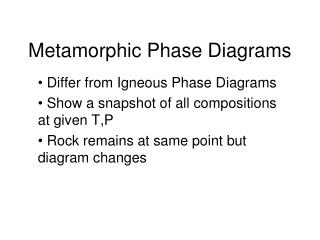# Metamorphic Phase Diagrams - PowerPoint PPT PresentationDownload PresentationMetamorphic Phase Diagrams

Metamorphic Phase DiagramsDownload Presentation## Metamorphic Phase Diagrams

- - - - - - - - - - - - - - - - - - - - - - - - - - - E N D - - - - - - - - - - - - - - - - - - - - - - - - - - -
##### Presentation Transcript

1. Metamorphic Phase Diagrams Differ from Igneous Phase Diagrams Show a snapshot of all compositions at given T,P Rock remains at same point but diagram changes

2. Phase Diagram for Water

3. The Phase Rule (Gibbs, 1928) Phases (Distinct Materials or States) + Degrees of Freedom (Independent Variables) = Components + 2

4. Phase Rule and Phase Diagrams

5. Phase Diagram for Al2SiO5

6. Phase Diagram for Ice

7. Phase Diagram of Silica

8. Degrees of Freedom • Pressure • Temperature • PH2O • PCO2 • pH • Oxygen fugacity • Eh

9. Simplifying Degrees of Freedom • Generally ignore pH, Eh, Oxygen fugacity for most rocks • Important for sulfide systems • Usually care about T,P • Assume PH2O = Ptotal for silicates, PCO2 = 0 • Assume PCO2 = Ptotal for carbonates, PH2O = 0 • Assume PH2O = 0 and PCO2 = 0 in some cases

10. Simplifying Degrees of Freedom • P + F = C + 2 • If F = 2, then P = C • Number of phases = number of components • Components = SiO2, Al2O3, Fe2O3, FeO, CaO, MgO, Na2O, K2O, TiO2, Cr2O3, MnO, BaO, SrO, P2O5, H2O, CO2, F, Cl

11. Simplifying Components • We can plot a maximum of three components using triangle diagrams • Ignore SiO2(excess), H2O (excess or 0), CO2 (excess or 0) • Assume P2O5 goes into apatite, Na2O into albite, TiO2 into rutile or ilmenite, Cr2O3, into chromite, F into fluorite or apatite, Cl into halite • Include MnO with FeO, BaO and SrO with CaO, Fe2O3 with Al2O3 or FeO, Cl, F with OH • Subtract major elements as necessary

12. Simplifying Components • Components reduced to Al2O3, FeO, CaO, MgO, K2O • ACF graphs Al2O3, CaO, (FeO+ MgO) • A’KF graphs Al2O3, (FeO + MgO), K2O • AFM graphs Al2O3, FeO, MgO, K2O in a tetrahedron • SiO2 – CaO – MgO used for carbonate systems • Plot Molar amounts, not weights

13. Metamorphic Phase Diagrams • Tie lines denote two coexisting phases • Triangular subfields denote three coexisting phases • Phase Diagram is snapshot of mineral combinations under given T,P conditions • Evolution tracked by changes in diagrams • Not really interested in amounts

14. Carbonate Rocks at 450C

15. Metamorphic Phase Diagrams • Normally F = 2, C = 3, P = 3 • On a reaction curve, F = 1, P = 4 • How to get 4 Phases Together: • New Phase Appears in Middle of Field • New Phase Appears on Tie Line • Tie Line Breaks and New One Forms • Changes in Metamorphism • New Minerals Appear • Old Minerals Disappear • Compatibilities Shift

16. Carbonate Rocks at Low T

17. 400 C: New Phase on Tie Line(Quartz + Brucite -> Talc)

18. 450 C: One Tie Line Replaced by Another(Quartz + Dolomite -> Calcite + Talc)

19. ACF Diagram • Probably most versatile and instructive diagram • A = (Al2O3 + Fe2O3) – (Na2O, + K2O) • Subtract Al2O3 in K-spar and albite • C = CaO - 3.3 * P2O5 • Subtract Ca in apatite • F = FeO + MgO + MnO

20. The ACF Diagram

21. A’KF Diagram • A’ = (Al2O3 + Fe2O3) – (Na2O, + K2O) – variable Ca (epidote, garnet, anorthite) • K = K2O • F = FeO + MgO + MnO – amount in diopside or hornblende • Distinguishes K-feldspar and micas

22. The A’KF Diagram

23. ACF and A’KF Diagrams are often paired

24. AFM Diagram • Graphs Al2O3, FeO, MgO, K2O • Cross-section through a tetrahedron • Used where MgO and FeO don’t fully substitute • Must include K2O because of micas • A = (Al2O3-3K2O)/(Al2O3-3K2O+FeO+MgO) • M = MgO/ (FeO + MgO) • F = FeO/ (FeO + MgO)

25. AFM Diagram

26. AFM Diagram

27. CaO-MgO-SiO2 Diagram